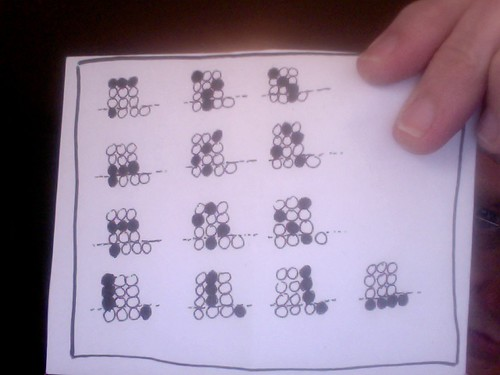## Archive for the ‘Uncategorized’ Category

### yet another test post

January 4, 2011February 14, 2010

z:= x+yi
w:= a+bi

zw = (xa-yb) + (xb+ya)i

u:= xa-yb
v:= xb+ya$f: \Bbb C \rightarrow M_2(\Bbb R)$$f(p+qi) := \begin{pmatrix} p & q \\ -q & p\end{pmatrix}$$f(z)f(w) = \begin{pmatrix} x & y \\ -y & x\end{pmatrix} \begin{pmatrix} a & b \\ -b & a \end{pmatrix} = \begin{pmatrix} xa-yb & xb+ya \\ -ya-xb & xa-yb \end{pmatrix} = \begin{pmatrix} u & v \\ -v & u\end{pmatrix} = f(zw)$

f(z)+f(w) = f(z+w) trivially.

January 5, 2010

(:={
0:=}

()${0 \choose 0} = 1$

(),(a)${1\choose0}=1, {1\choose1}=1$

(), (a) (b), (a,b)${2 \choose 0} = 1, {2\choose 1} = 2, {2\choose 2} = 1$

(),(a)(b)(c),(b.c)(a.c)(a.b),(a.b.c)${3\choose0}=1,{3\choose1}=3,{3\choose2}=3,{3\choose3}=$

### Archive

November 23, 2009

As I Was Saying
The Natural Log: Eli Maor plugged.
In The Arena: “inflexible knowledge” at KTM & Lefty on Devlin.
exp & log
Math Newsbite: “Square root day”.
Introduction to Logarithmic Functions
The Veil of Maya: simple, compound, & continuous interest.
When There Is No Peace: links.
Stretching A Point: doubling time etcetera.
Why Was I Not Informed?: MT@P, Spirograph.
Catherine Nails It: 21st C. skills.
Remarks Toward The Dissolution Of This Blog: review.
Herculean Sense-Making: Garelick on discovery learning.
More Review
3.14
Last Post

### Catherine Nails It

March 7, 2009

Check out “why 21st century skills” at KTM.

### Stretching A Point

March 6, 2009

The function$[t \mapsto A_0 2^{t/K}]$ is the exponential function with doubling time K and initial value A_0. I’ve capitalized the constants (as I usually try to do); the units of K are (of course) those of t (which can be arbitrary; our assumption yesterday that t would be in years was for yesterday’s material only). Thus, for example, a population of one million (at time zero; look at what happens in the exponential function under consideration; A_0 is a million) that doubles every 32 years would be represented by f(x) = 10^6 * 2^(t/32). Now what?

Well, I suppose one should plot the co-ordinate pairs having t equal to (say) 0, 32, and 64 and sketch the curve; look at the calculator; that kind of thing. But we’ll assume everybody gets it. You probably oughta be looking at a source with graphs in it if you don’t already understand this theory pretty well; I’m pretty helpless with online graphics.

Now, one could define the same exponential function in terms of its “tripling time” instead of doubling time; indeed putting f(x) = 3*10^6 in our example yields 3 = 2^(t/32); taking logs (base two) on both sides gives us t/32 = log_2 (3) and hence t = 32 log_2 (3)…about 50.72 years. So g(x) = 10^6 * 3^(t/50.72) is essentially the same function as f (to make g exactly equal to f, replace “50.72” with the exact value (the “formula”; for some reason students tend to believe that decimals are more “exact” than the algebra they approximate [and have a hard time letting go of this idea]).

What I have in mind is some alternative universe (or future class) where I would hold back on introducing the “exponential growth rate” for at least one section and have students work on exercises about doubling times (and half-lives; I intended to have mentioned by now that when K is negative one has an exponential decay rather than an exponential growth and that -K is then called the half-life of the quantity being measured [usually in this context, “number of molecules of a certain radioactive isotope”]). Things are already hard enough to assimilate without some weird number unknown to elementary mathematics being thrown into the mix.

Passing back and forth between the bases of 2 and 3 could, at least for some students, make it easier to see what’s going on in transforming to the base e… and this “interchangiblity” of the bases seems to be something that students typically seem not to quite “get”. Anyhow, the graphs I’m thinking of here—and’ll have on the board in a few hours—would be w(x)= 2^x and h(x)= 3^x (on the same co-ordinate axes). If we think of “beginning at” 2^x and “going to” 3^x, we’re applying a transformation. This one looks like a horizontal “shrinking” (note in particular that the fixed point is on the y-axis). And indeed it is. Assuming this for a moment, we can conclude that the functions w and h are related by h(x) = w(Lx) for some “scaling factor” L.

I’ll interupt myself to remark that we know this from work in the first week of the course… adds and subtracts are shifts, multiplies and divides are scalings, and sign changes are reflections; things that happen to y go up and down and things that happen to x go side-to-side; finally (and most trickily) such side-to-side moves occur “backward” in the sense that, for example, adding a positive number to the “argument” (replacing f(x) with f(x+3), say) calls for subtracting from the x co-ordinates in computing points on the “new” graph (shifting left by three units in our example).

Returning to our exponential functions, we’ve used geometry to see that 3^x can be written as 2^(Lx) for some L (the scaling factor). Some “simple” algebra (perfectly natural and straightforward once one has done the exercises) tells us that L = log_2 (3) here; indeed$2^{\log_2 (3)t} = (2^{\log_2(3)})^t = 3^t$. It would hardly be an exaggeration to say this is what log_a is for: determining the scaling factors for passing from one exponential function to another. Gotta go; see you soon I hope.